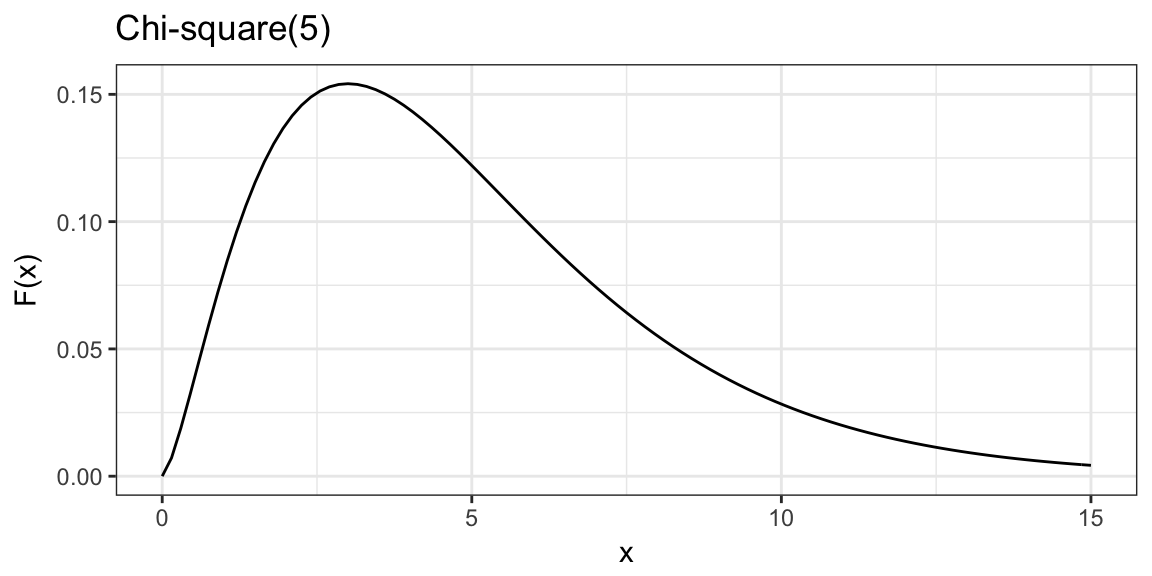# Finish last time’s slides

The K-S test is only appropriate for continuous distirbutions (the hypothesized distribution is continuous).

But what about if our hypothesis if for a discrete distribution, e.g.:

• Discrete Uniform
• Bernoulli
• Poisson

## Setting

Population: $$Y \sim$$ some discrete population distribution with p.m.f $$p(y) = P(Y = y)$$

Sample: n i.i.d from population, $$Y_1, \ldots, Y_n$$

Parameter: Whole p.m.f

Hypotheses: $$H_0: P(Y = y) = p_0(y)$$, versus $$H_A: P(Y = y) \ne p_0(y)$$

## Sample estimate of the p.m.f

The discrete sample based estimate of the probability mass function:

$\hat{p}(y) = \frac{1}{n} \sum_{i = 1}^{n} \pmb{1} \left\{ Y_i = y \right\}$

## The Chi-square Goodness of Fit test

Chi-square goodness of fit compares the estimated p.m.f to the hypothesized one.

Pearson’s Chi-square statistic: $X(p_0) = \sum_y \frac{n\left( \hat{p}(y) - p_0(y) \right)^2}{p_0(y)}$

Under null hypothesis: $$X(p_0)$$ converges in distibrution (as n goes to infinity) to $$\chi^2$$ with $$k-1$$ degrees of freedom

$$k=$$ number of possible values for $$Y$$.

## An alternative presentation

• $$j = 1, \ldots, n$$ indexes possible values/categories for $$Y$$
• $$O_j$$ be the observed number of values in category $$j$$
• $$E_j = np_0(j)$$ be the expected number of values in category $$j$$, based on the hypothesized distribution.

Pearson’s Chi-square statistic: $X(p_0) = \sum_{j = 1}^{k} \frac{(O_j - E_j)^2}{E_j}$

## Example: Dice rolling

I rolled a die 60 times and recorded how many times I got each side: 1, 2, 3, 4, 5, 6

Question: Is the die fair? That is, is $$p_0(j) = 1/6$$ for $$j = 1, 2, 3, 4, 5, 6$$?

1 2 3 4 5 6
$$O_j$$ 20 11 6 7 6 10

## Rejection region

Reject $$H_0$$ for $$X(p_0) > \chi^2_{(k-1)} (1-\alpha)$$

Rejection region always to the right, p-values always area to right. Why? We usualy are only interested in evidence of poor fit (not evidence of extra good fit)

## Example: Dice rolling cont.

Compare to $$\chi_{(5)}^2$$qchisq(0.95, df = 5)
##  11.0705
1 - pchisq(chi_sq, df = 5)
##  0.01438768

## In R:

rolls
##   6 5 2 2 6 2 1 1 5 1 2 2 1 1 3 4 5 1 2 4 1 6 6
##  2 4 1 6 6 1 6 1 1 6 1 3 1 3 4 1 1 5 3 4 2 5 1
##  6 3 1 4 3 2 5 1 6 2 1 4 1 2
chisq.test(table(rolls), p = rep(1/6, 6))
##
##  Chi-squared test for given probabilities
##
## data:  table(rolls)
## X-squared = 14.2, df = 5, p-value = 0.01439

## Estimation of parameters

If the null hypothesis doesn’t completely specify the distribution $$p_0$$, but specifes a family of distributions, $$p_0(\theta_1, \theta_2, \ldots, \theta_d)$$ where the $$\theta$$ are unknown parameters.

You can still use the Chi-square test with some modification

1. Estimate the parameters $$\theta_1, \theta_2, \ldots, \theta_d$$
2. Find $$E_j$$ based on estimated parameters and $$p_0$$.
3. Compute Pearson’s $$\chi^2$$ statistic as usual
4. Compare statistic to a $$\chi^2$$ with $$k - d - 1$$ degrees of freedom, where $$d$$ is the number of parameters that were estimated.

## Example: Poisson

I counted the number of passengers in $$n = 40$$ vehicles passing through an intersection.

Question: Is the number of passengers per vehicle distributed according to a Poisson distribution?

0 1 2 3 4 5 6
$$O_j$$ 6 11 11 8 3 0 1
mean(passengers)
##  1.875

## Example: Poisson

0 1 2 3 4 5 6
$$O_j$$ 6 11 11 8 3 0 1
p_0 <- dpois(0:6, lambda = mean(passengers))
(E <- p_0 * n)
##   6.1341987 11.5016225 10.7827711  6.7392319
##   3.1590150  1.1846306  0.3701971
# For 7+ category
(E_7 <- (1 - sum(p_0)) * n)
##  0.1283331

## Example: Poisson

Test statistic:

$\frac{(6 - 6.13)^2}{6.13} + \frac{(11 - 11.5)^2}{11.5} + \ldots + \frac{(0 - 0.13)^2}{0.13} = 2.66$

Compare to $$\chi^2_{k - d - 1}$$

1 - pchisq(X, df)
##  0.8504434

## Other points

• For binary data, the $$X(p_0)$$ statistic is equal to the square of the Z-statistic for testing a hypothesis regarding a binary proportion.

Therefore, for two sided hypothesis testing $$H_0: p = p_0$$ vs $$H_A: p \ne p_0$$, the $$\chi^2$$ test and the z-test give the exact same result.

• The $$\chi^2$$ statistic has an asymptotic $$\chi^2$$ distribution.

Therefore this test is approximate: the test is asymptotically exact.

The approximation is generally considered appropriate when $$E_j > 5$$ for all $$j$$.

## Next time

Starting two sample inference…